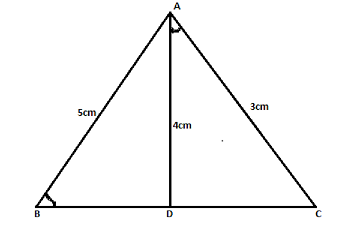Courses
Courses for Kids
Free study material
Free LIVE classes
MoreLIVE
Join Vedantu’s FREE Mastercalss

# In the adjoining figure D is a point on BC such that, ∠ABD = ∠CAD. If AB = 5cm, AD = 4cm and AC = 3cm. Find A (∆ACD) : A (∆BCA).Verified
362.1k+ views
Hint: Visualizing the given data in the question draws an appropriate figure. According to the properties of triangles, observe similarities between the two triangles in order to establish a relation between their angles or sides.Given data,
∠ABD = ∠CAD. AB = 5cm, AD = 4cm and AC = 3cm.

Let us compare ∆ABC and ∆CAD
∠ACB = ∠ACD (common angle, from the figure)
The Third Angle theorem states, if two angles of a triangle are congruent to two angles in another triangle, then the third pair of angles are also congruent.
Hence, the third angles of ∆ABC and ∆CAD are congruent
⟹∠CAB = ∠CDA
Using AAA rule, we can say, ∆ABC ~ ∆CAD
(AAA rule, which states if 3 angles are congruent between two triangles then the triangles are similar i.e. of the same shape which typically means the ratio of their areas, ratios of corresponding angles are equal)
Also, the ratio of areas of similar triangles is equal to the ratio of squares of corresponding sides.
⟹ $\dfrac{{{\text{A}}\left( {\Delta {\text{ACD}}} \right)}}{{{\text{A}}\left( {\Delta {\text{BCA}}} \right)}} = {\text{ }}\dfrac{{{\text{A}}{{\text{D}}^2}}}{{{\text{A}}{{\text{B}}^2}}}$
From given AD = 4cm, AB = 5 cm
⟹ $\dfrac{{{\text{A}}\left( {\Delta {\text{ACD}}} \right)}}{{{\text{A}}\left( {\Delta {\text{BCA}}} \right)}} = {\text{ }}\dfrac{{{4^2}}}{{{5^2}}}$
⟹ $\dfrac{{{\text{A}}\left( {\Delta {\text{ACD}}} \right)}}{{{\text{A}}\left( {\Delta {\text{BCA}}} \right)}} = {\text{ }}\dfrac{{16}}{{25}}$

Note: In order to solve these types of questions the key is to make a precise figure which depicts all the given data. Then observe for similarities using properties of triangles. Keep in mind the AAA rule only states that the triangles are similar, they may or may not be congruent.
Last updated date: 29th Sep 2023
Total views: 362.1k
Views today: 6.62k